# Plane figures and shapes

Welcome to Class !!

In today’s Mathematics class, We will be discussing Plane Figures and Shapes. We hope you enjoy the class!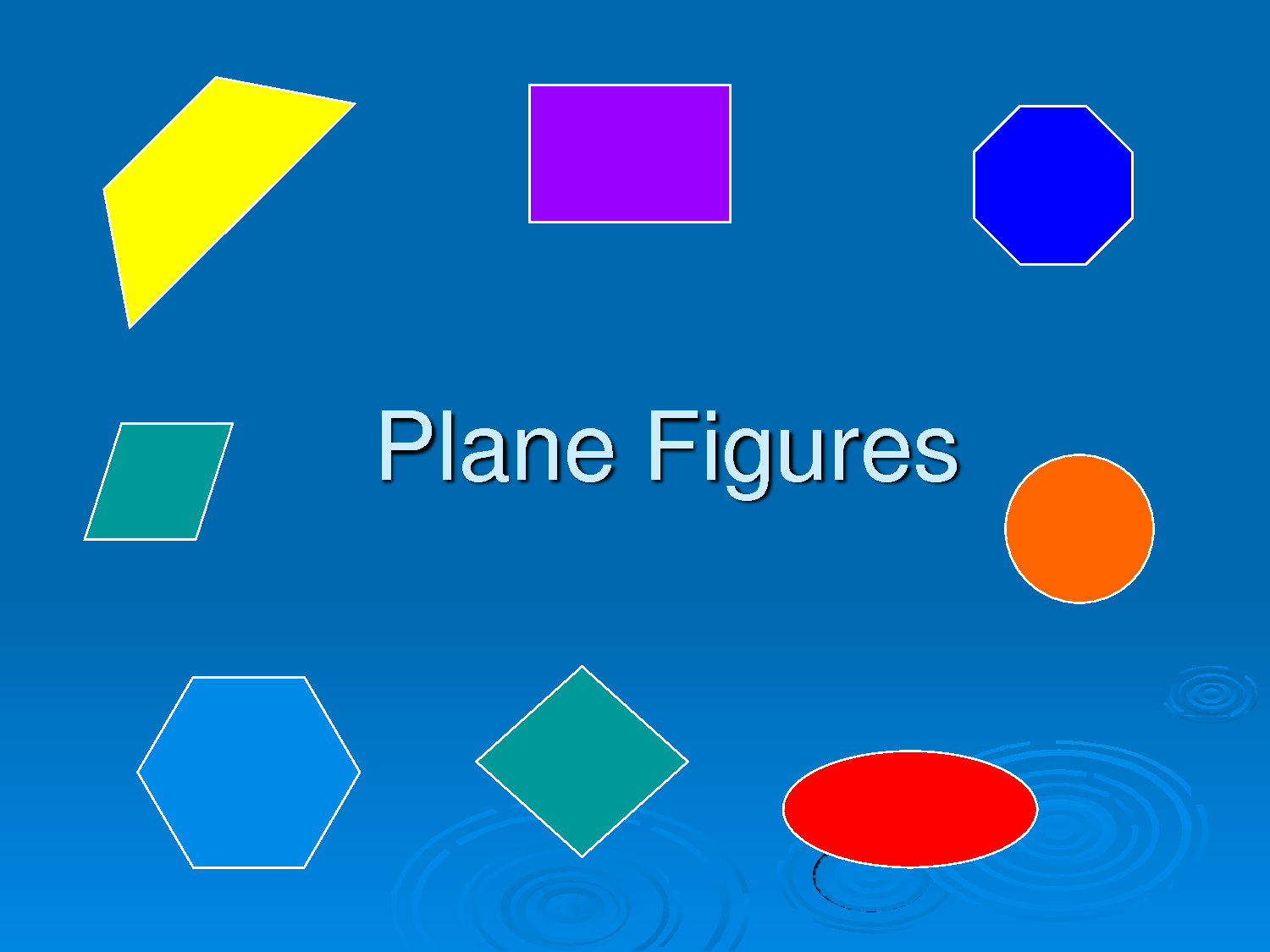Any four-sided shape is a Quadrilateral.

But the sides have to be straight, and it has to be 2-dimensional.Properties

Four sides (edges)

Four vertices (corners)

The interior angles add up to 360 degrees:Try drawing a quadrilateral, and measure the angles. They should add to 360°

There are special types of quadrilateral: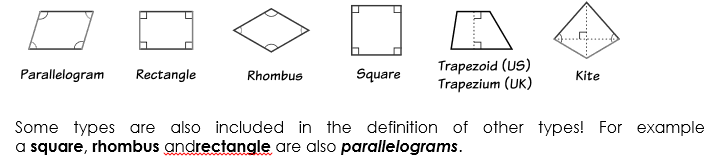Some types are also included in the definition of other types! For example, a squarerhombus, and rectangle are also parallelograms.

Let us look at each type in turn:

The Rectangle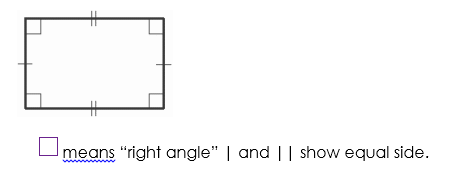A rectangle is a four-sided shape where every angle is a right angle (90°).

Also opposite sides are parallel and of equal length.

#### The Rhombus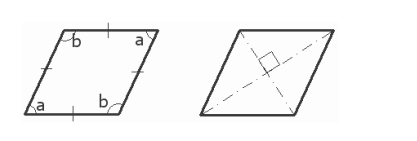A rhombus is a four-sided shape where all sides have equal length.

Also, the opposite sides are parallel and opposite angles are equal.

Another interesting thing is that the diagonals (dashed lines in the second figure) meet in the middle at a right angle. In other words, they “bisect” (cut in half) each other at right angles.

A rhombus is sometimes called a rhombus or a diamond.

#### The Squaremeans “right angle” | shows equal side.

A square has equal sides and every angle is a right angle (90°)

Also, the opposite sides are parallel.

A square also fits the definition of a rectangle (all angles are 90°), and a rhombus (all sides are equal length).

#### The ParallelogramA parallelogram has opposite sides parallel and equal in length. Also, opposite angles are equal (angles “a” are the same, and angles “b” are the same).

NOTE: Squares, Rectangles and Rhombuses are all Parallelograms!

#### The Trapezoid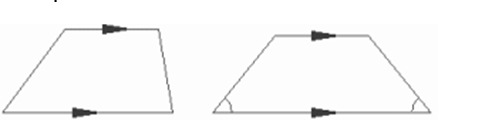A trapezoid (called a trapezium in the UK) has a pair of opposite sides parallel.

It is called an Isosceles trapezoid if the sides that aren’t parallel are equal in length and both angles coming from a parallel side are equal, as shown.

And a trapezium (UK: trapezoid) is a quadrilateral with NO parallel sides:

#### The KiteHey, it looks like a kite. It has two pairs of sides. Each pair is made up of adjacent sides that are equal in length. The angles are equal where the pairs meet. Diagonals (dashed lines) meet at a right angle, and one of the diagonal bisects (cuts equally in half) the other.

Note: The only regular quadrilateral is a square. So all other quadrilaterals are irregular.

The definition shows that squares are special rectangles. Thus all squares are also rectangles. However, some rectangles are not squares.

Parallelograms have two pair sides parallel. This is also true of squares, rectangles and rhombuses. Thus squares, rectangles and rhombuses are special parallelograms. However, there are many parallelograms which are not squares, rectangle or rhombuses.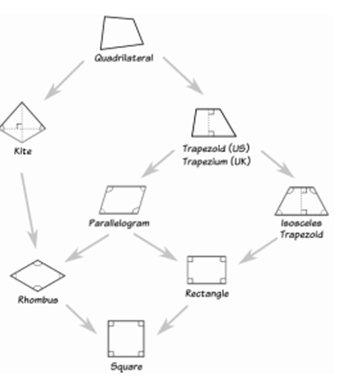Weekend Assessment

1. Name four shapes which are special examples of trapezium
2. Name three shapes which are special examples of parallelograms
3. Name two shapes which are special examples of kites.

We have come to the end of this class. We do hope you enjoyed the class?

Should you have any further question, feel free to ask in the comment section below and trust us to respond as soon as possible.

In our next class, we will be talking about Scale drawing of lengths and distances. We are very much eager to meet you there.

Are you a Parent? Share your quick opinion and win free 2-month Premium Subscription

Don`t copy text!